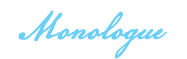# 原型链

### 含义

• 构造函数,原型,实例的关系,每个构造函数的原型都会有一个内部指针指向该构造函数,每个构造函数的实例都会有一个内部指针指向该构造函数的原型;如果把该构造函数的原型当做另外一个构造函数的实例,那么该实例就会有一个内部指针指向新的构造函数的原型对象,新的构造函数的原型对象会有一个内部指针指向新的构造函数,这样层层嵌套下去就形成了原型链
• 特别的一支原型链: 每个继承者的原型都是被继承者的实例,所以每个函数的原型都是Object的实例

### 图示

• 原型对象.constructor = 构造函数

• 实例.proto = 原型对象

• Function 是顶层构造器

• Object 是顶层原型### 代码

```function A() { this.a = true } A.prototype.getAProperty = function () { return this.a } function B() { this.b = false } B.prototype = new A() //此处的B的原型指向了A的构造函数 //可以手动把B的原型指向重新改回来 // B.prototype = { // constructor: B // } B.prototype.getBProperty = function(){ return this.b } let instance = new B() let res = instance.getAProperty() console.log(res) //true```